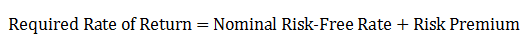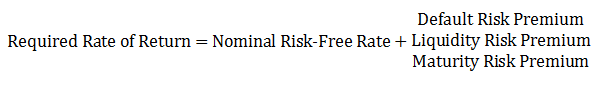# Interest Rate Equation

The required interest rate that an investor earns from an investment is made up of various components. The general interest rate equation is expressed below:The nominal risk-free rate itself is expressed as the sum of real-risk free rate and inflation premium.

It is important to understand the difference between the nominal and real risk-free interest rates. Nominal interest rates are what we observe everyday as published by banks and other financial institutions. For example, when a T-bill pays 6% interest that is the nominal risk-free interest rate. It already includes the premium for expected inflation. On the other hand, real interest rates take purchasing power parity into consideration. That’s the rate, which tells how much more you will be able to buy with your grown investment after one year.

So, the minimum that you expect from a risk-free investment is the nominal risk-free rate. Apart from that you will also expect a risk premium for various types of additional risks that you take by investment in a particular asset or security.

These risks come in many forms and a premium for each should be added to the risk-free rate to arrive at the required rate of return from an investment. The important types of risk are:

• Default Risk: The risk that the borrower will be unable to meet its payment obligations or keep up with the terms of payments.
• Liquidity Risk: The risk that the investment is less liquid and that the investor may have to sell the bond at a price lower than the expected price.
• Maturity Risk: The longer the maturity of a bond, the more volatile will be its price.

A premium for each of these risks is added to the nominal risk-free rate (such as T-bill rate) to arrive at the required rate of return. We can rewrite the interest rate equation as follows: